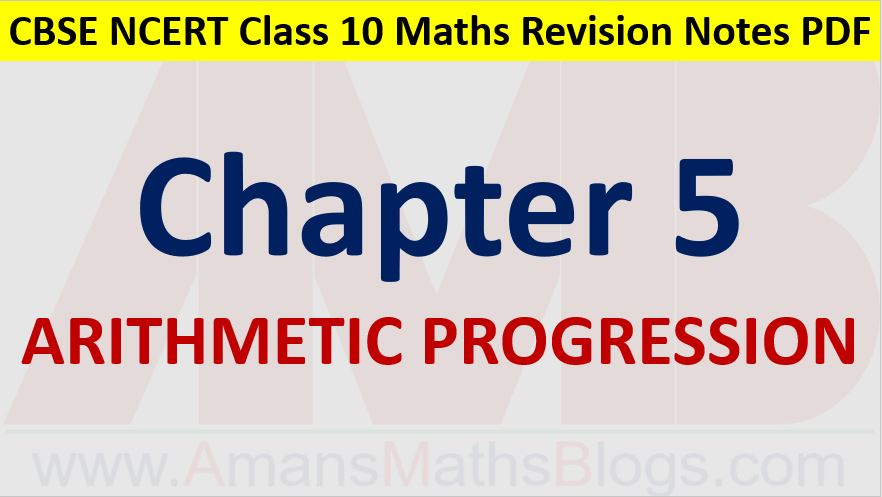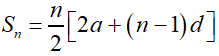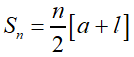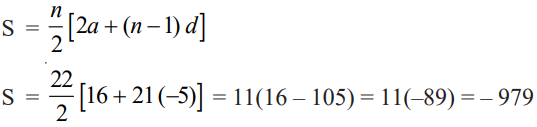Friday, October 22, 2021
Home > CBSE Class 10 > Arithmetic Progressions CBSE NCERT Notes Class 10 Maths Chapter 5 PDF

# Arithmetic Progressions CBSE NCERT Notes Class 10 Maths Chapter 5 PDFHi students, Welcome to AMBiPi (Amans Maths Blogs). In this article, you will get Arithmetic Progressions CBSE Notes Class 10 Maths Chapter 5 PDF. You can download this PDF and save it in your mobile device or laptop etc.

# Arithmetic Progressions CBSE Notes Class 10 Maths Chapter 5 PDF

## Arithmetic Progression

An arithmetic progression is a list of numbers in which each term is obtained by adding a fixed number to the preceding term except the first term. This fixed number is called the common difference of the AP and the common difference of AP can be positive, negative or zero.

If the sequence t1, t2, t3, …, tn-1, tn are in AP, then t2 – t1 = t3 – t2 = t4 – t3 = … = tn – tn-1 = d (common difference).

The sequence a, a + d, a + 2d, a + 3d, . . . represents an arithmetic progression where a is the first term and d the common difference. This is called the general form of an AP.

### Class 10 Maths Chapter 5 Examples 1:

Write first four terms of the AP, when the first term a = 10 and the common difference d = 10.

The arithmetic progression is

a, a + d, a + 2d, a + 3d, . . . ⇒ 10, 20, 30, 40, …

### Class 10 Maths Chapter 5 Examples 2:

Check whether the sequence 2, 4, 8, 16, . . . is in AP or not.

4 – 2 ≠ 8 – 4. Thus, the given sequence is NOT in AP.

### Class 10 Maths Chapter 5 Examples 3:

For the AP, 0.6, 1.7, 2.8, 3.9, . . ., write the first term and the common difference.

The first term of the AP is a = 0.6 and the common difference is d = 1.7 – 0.6 = 1.1.

## nth Term of General Term of an AP

If the sequence t1, t2, t3, …, tn-1, tn are in AP, then the general term of the AP is tn = a + (n – 1)d, where a is the first term (a = t1) and d is the common difference (d = t2 – t1 = t3 – t2 = … etc).

## nth Term of General Term of an AP from Last

Let a be the first term (a = t1) and d be the common difference (d = t2 – t1 = t3 – t2 = … etc) of an AP having total N terms. Then, the nth term from the end or last of the AP is the (N – n + 1)th term from the beginning of the AP.

### Class 10 Maths Chapter 5 Examples 4:

Find the 10th term of the AP : 2, 7, 12, . . .

Given that a = 2, d = 7 – 2 = 5.

Since tn = a + (n – 1)d

⇒ t10 = 2 + (10 – 1)(5) = 2 + 9 × 5 = 2 + 45 = 47

### Class 10 Maths Chapter 5 Examples 5:

Check whether 301 is a term of the list of numbers 5, 11, 17, 23, . . .

tn = a + (n – 1)d

⇒ 301 = 5 + (n – 1)6

⇒ 301 = 5 + 6n – 6

⇒ 301 = 6n – 1

⇒ 6n = 302

⇒ n = 151/3 (Not Natural number).

Thus, 301 is not a term of the given sequence.

### Class 10 Maths Chapter 5 Examples 6:

Which term of the AP : 21, 18, 15, . . . is – 81?

Given that a = 21, d = 18 – 21 = -3.

Since tn = a + (n – 1)d

⇒ –81 = 21 + (n – 1)(-3)

⇒ –81 = 21 – 3n + 3

⇒ 3n = 24 + 81 = 105

⇒ 3n = 105

⇒ n = 105 / 3 = 35

## Sum of n Terms of an AP

Let a be the first term (a = t1) and d be the common difference (d = t2 – t1 = t3 – t2 = … etc) of an AP having total n terms. Then, the sum of n terms of AP isIf the last term or nth term of the AP is known, then the sum of n terms of AP isThe sum of first n natural numbers is S = 1 + 2 + 3 + … + n = n(n + 1)/2.

### Class 10 Maths Chapter 5 Examples 7:

Find the sum of the first 22 terms of the AP : 8, 3, –2, . . ..

Given that a = 8, d = 3 – 8 = –5, n = 22.### Class 10 Maths Chapter 5 Examples 8:

Find the sum of the first 1000 positive integers.

Required sum S = 1 + 2 + 3 + … + 1000 = 1000(1000 + 1)/2 = 500 × 1001 = 500500.

Click below to get CBSE Class 10 Maths Chapter wise Revision Notes PDF.

Chapter NoChapter name
Chapter 1 :Real Numbers CBSE Class 10 Maths Revision Notes
Chapter 2 :Polynomials CBSE Class 10 Maths Revision Notes
Chapter 3 :Pair of Linear Equations in Two Variables CBSE Class 10 Maths Revision Notes
Chapter 4 :Quadratic Equations CBSE Class 10 Maths Revision Notes
Chapter 5 :Arithmetic Progression CBSE Class 10 Maths Revision Notes
Chapter 6 :Triangles CBSE Class 10 Maths Revision Notes
Chapter 7 :Coordinate Geometry CBSE Class 10 Maths Revision Notes
Chapter 8 :Introduction to Trigonometry CBSE Class 10 Maths Revision Notes
Chapter 9 :Some Applications of Trigonometry CBSE Class 10 Maths Revision Notes
Chapter 10 :Circles CBSE Class 10 Maths Revision Notes
Chapter 11 :Constructions CBSE Class 10 Maths Revision Notes
Chapter 12 :Area Related to Circles CBSE Class 10 Maths Revision Notes
Chapter 13 :Surface Areas & Volumes CBSE Class 10 Maths Revision Notes
Chapter 14 :Statistics CBSE Class 10 Maths Revision Notes
Chapter 15 :Probability CBSE Class 10 Maths Revision Notes
AMBiPi
error: Content is protected !!